Ever heard of the Goal Seek feature in Excel? It is a very nice feature wherein it takes out the guesswork for you and determines the input value needed to achieve a specific goal. For example, you have a goal / result in mind, but you are unsure what the starting amount should be for an investment. Let us use Excel Macros to execute the Goal Seek feature!

Make sure your Excel has the Developer Tab enabled following this tutorial.

I explain how you can do this below step by step!

What does it do?

Executes Goal Seek in Excel

Copy Source Code:

```
'Make sure the worksheet is selected to execute the Goal Seek on
Sub GoalSeekVBA()

Dim TargetGoal As Long

'Get the target value from the user
TargetGoal = InputBox("Enter the target value", "Enter Goal")

'Make sure to change the cell that you want to be changed with the goal
ActiveSheet.Range("E9").GoalSeek _
Goal:=TargetGoal, _
ChangingCell:=Range("A9")

End Sub

```

Final Result: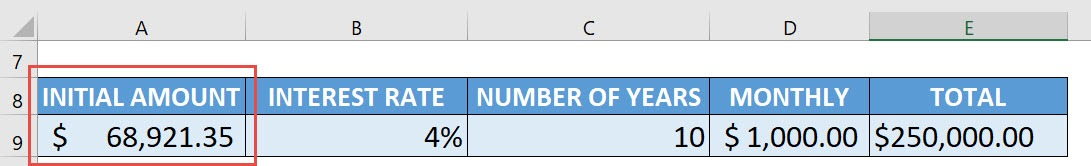Exercise Workbook:This is our scenario. We have a formula calculating our target goal:

• The initial amount is \$5000
• Interest rate is 4%
• Number of years is 10
• Monthly additional investment is \$1000
• With these parameters, after 10 years, your investment will be equivalent to \$154,703.97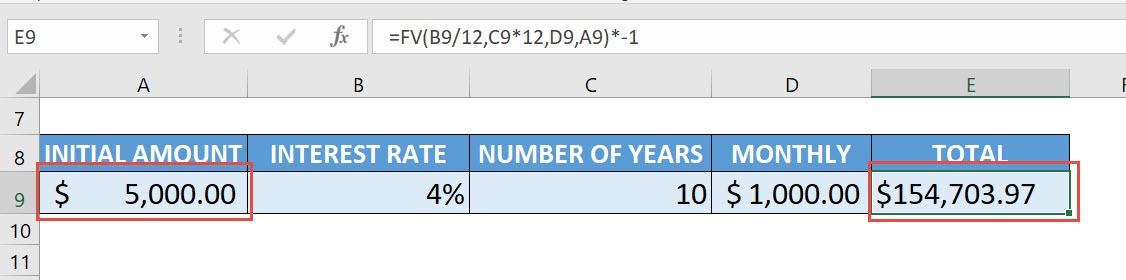Let us now assume that, given we want to achieve a goal of \$250,000. What will our initial amount be?

Take note of the following:

• Initial Amount – Cell A9
• Total Amount (Goal) – Cell E9

STEP 1: Go to Developer > Code > Visual Basic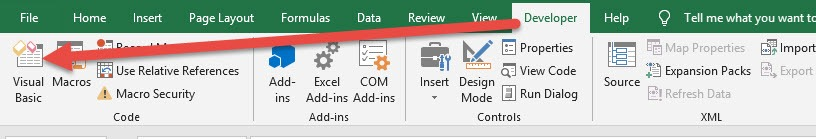STEP 2: Paste in your code and Select Save. Close the window afterwards.

Do take note that we are referencing these two cells in the code:

• Initial Amount – Cell A9
• Total Amount (Goal) – Cell E9

What goal seek will do, is it will adjust the initial amount (Cell A9), to achieve the target goal that you specify (Cell E9) which is \$250,000.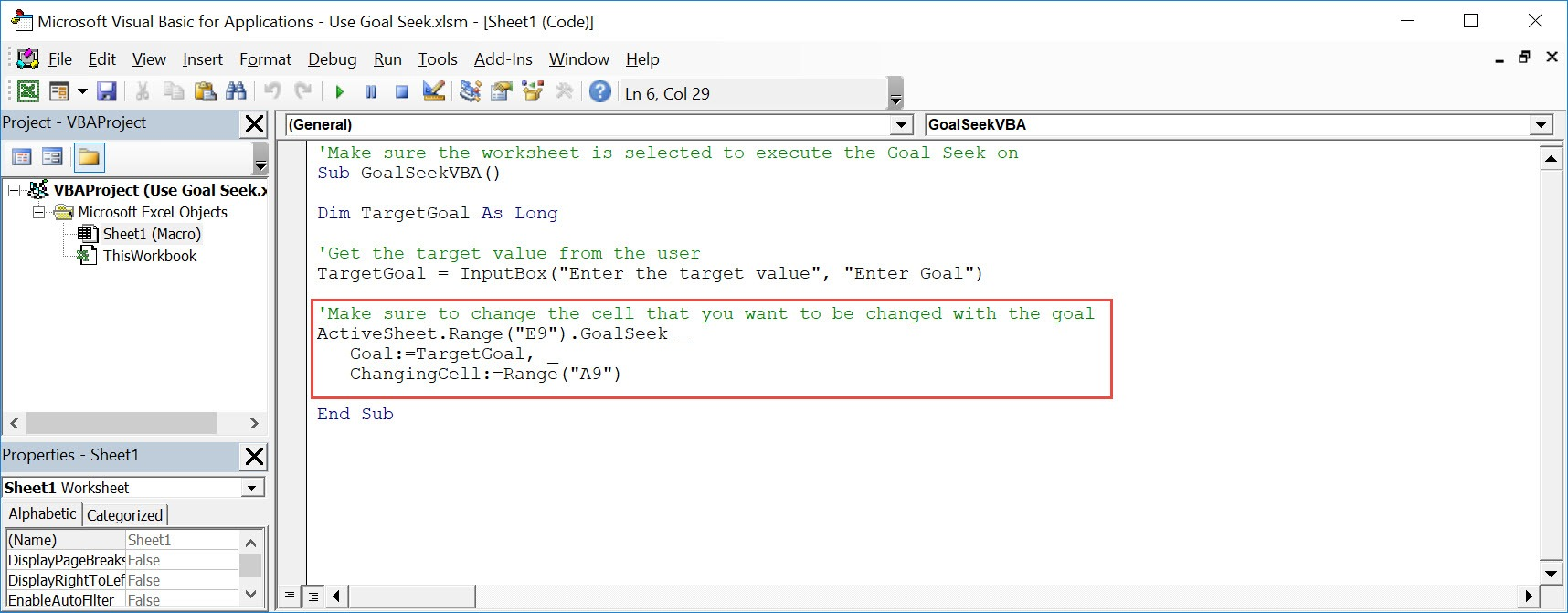STEP 3: Let us test it out!

Open the sheet containing the data. Go to Developer > Code > Macros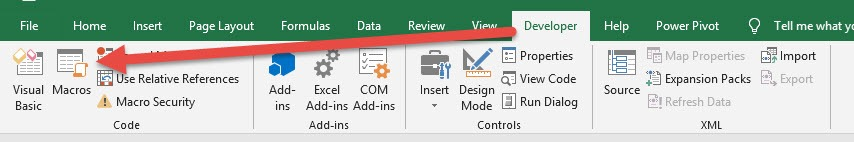Make sure your macro is selected. Click Run.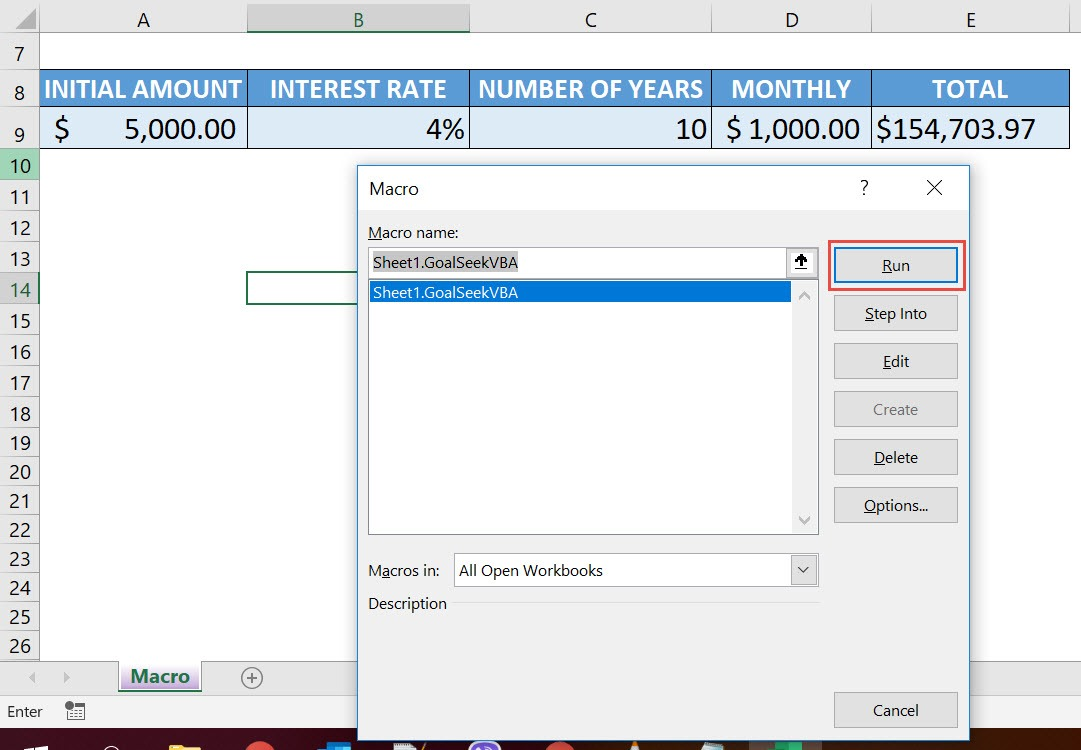Type in the target value of \$250,000. See how the initial amount will change.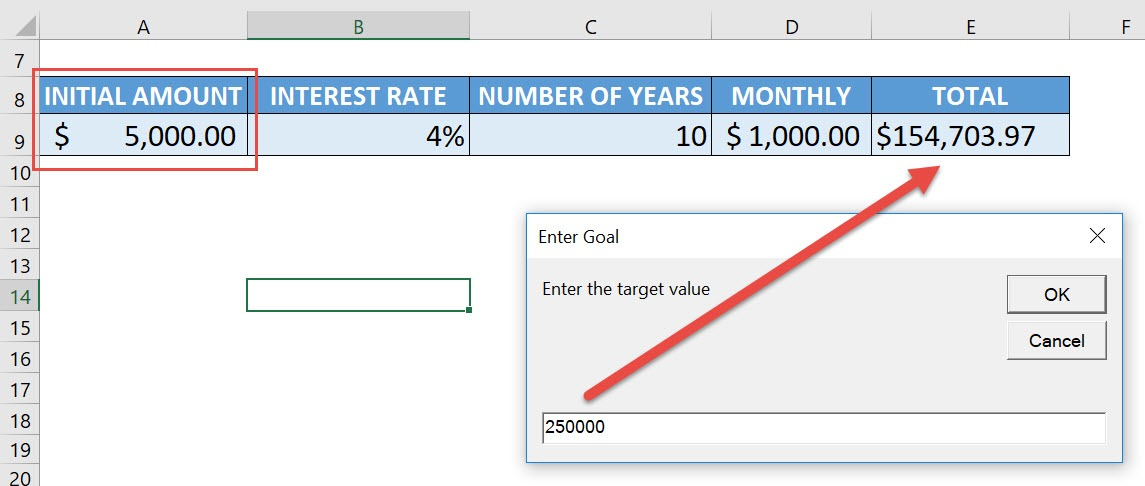With just one click, Goal Seek computed that you need an initial amount of \$68,921.35 to achieve your goal of \$250,000!How to Use Goal Seek Using Macros In Excel